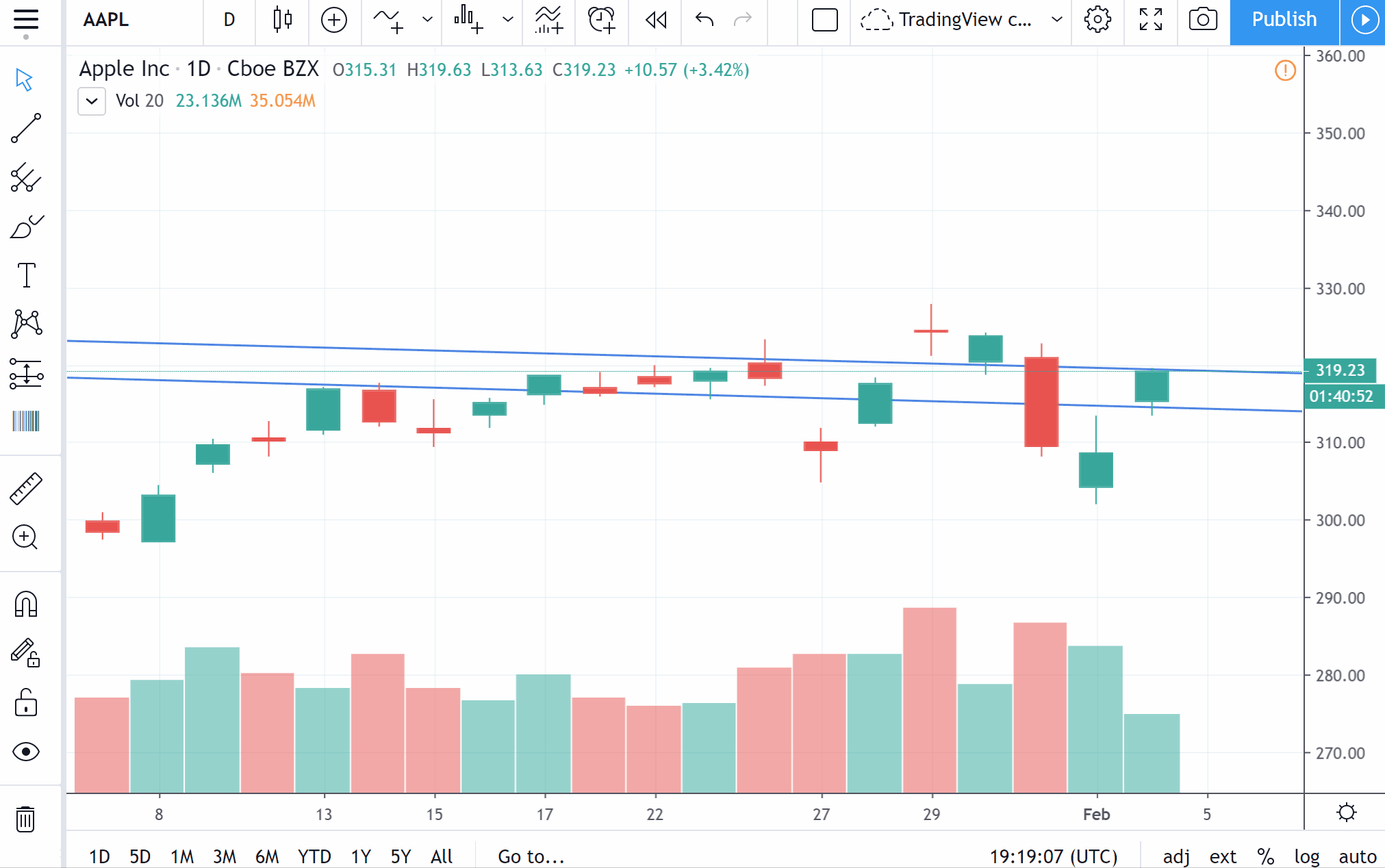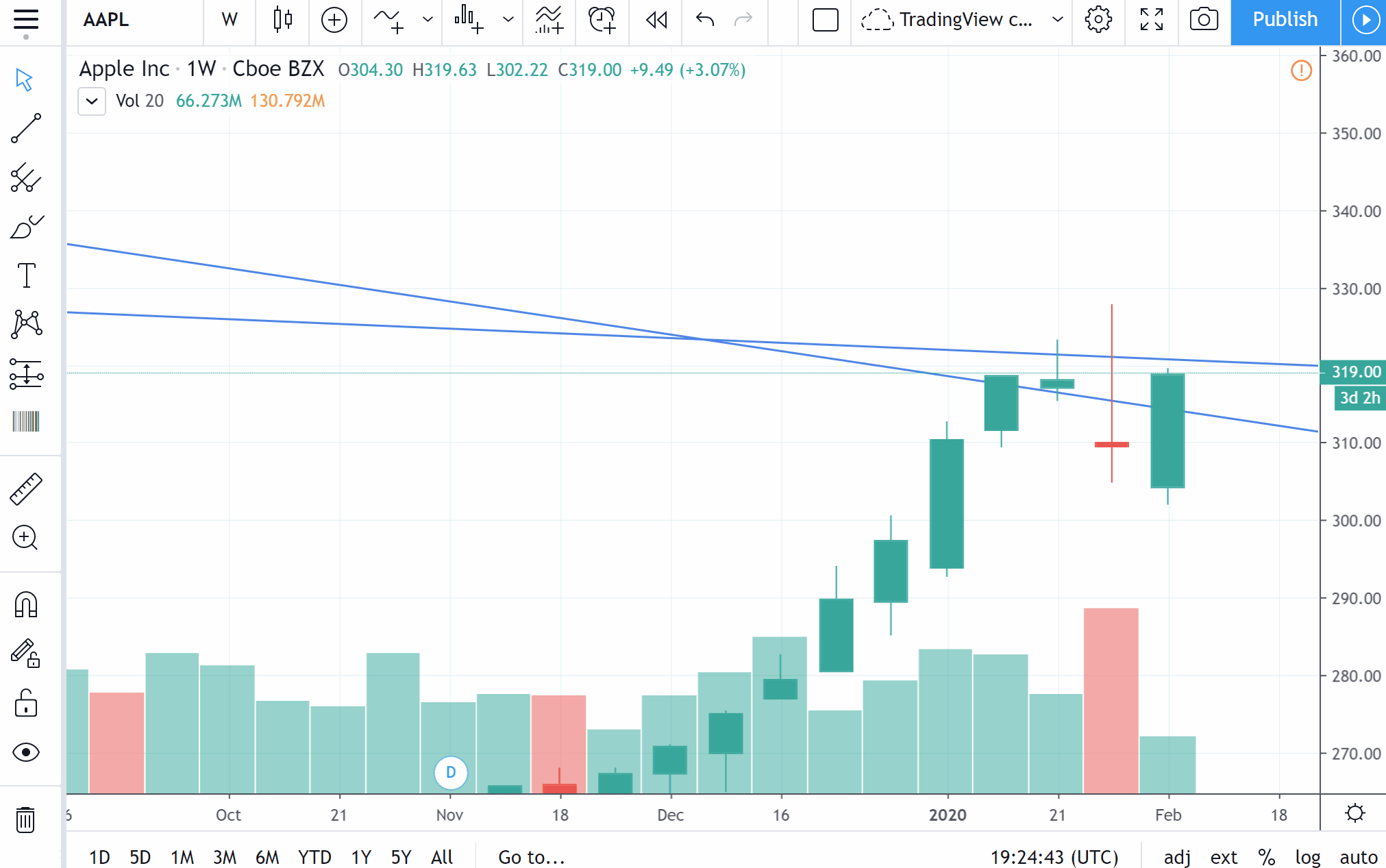# Lines become non-parallel when switching interval

Drawings that are parallel when applied to an interval, might be non-parallel on another one — this is expected.

For example, we added 2 parallel lines via Trend Line drawing tool to the chart with 1-day interval:Then we change the interval to 1 week and see that the lines are crossing now.This is due to the fact that there might be a different number of bars between the fixed points of the lines. As a result, the length of the line projection between the points on the time axis changes, while projection length on the price axis does not change. Thus, the line angle changes, according to its coordinates. As the coordinates differ, the angles might also change. So, when you change the interval, lines might become non-parallel.